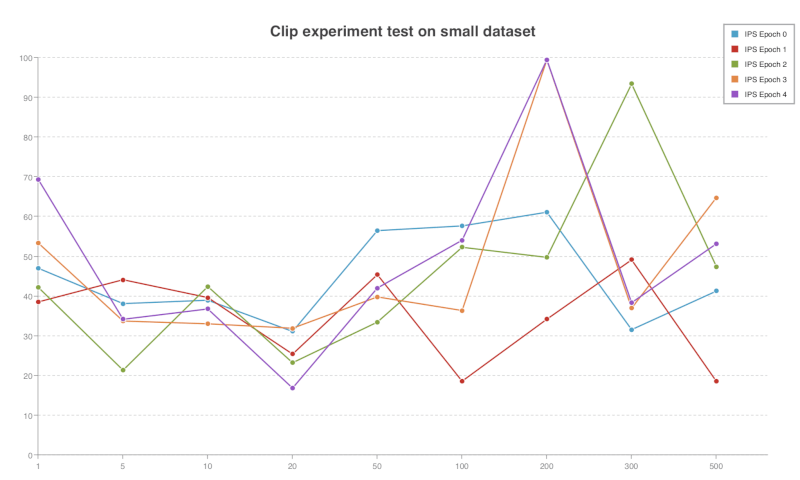Weekly ReportJinning Li Aug 02, 2018

Weekly Report 

Jinning, 08/02/2018

Propensity weighted BCE loss

Run experiment with both propensity weighted or not. All the two result are based on same hyperparameters:

• Non-Propensity (weight: 1) Epoch 10: IPS: 54.5463082902, IPS_std: 2.943

• propensity (weight: 1/propensity) Epoch 10: IPS: 55.1079999611, IPS_std: 6.328

Intuition:

Introducing propensity can improve the performance of LR model slightly. Overfitting will probably happen at epoch 10. It’s possible that propensity can reduce the bias and relieve overfitting.

Clip Experiment

Clip propensity weighted BCE loss. This means the weight applied to BCE loss is not simply $\frac{1}{p}$, but $\min\{\frac{1}{p}, c\}$, where $c$ is a constant. In my experiment, $c$ is selected as 1, 5, 10, 20, 50, 100, 200, 300, 500.

When $c=1$, this is equivalent to unweighted BCE. When $c=500$, since $\frac{1}{p}$ is less than $500$, this is equivalent to $\frac{1}{p}\times loss$.

Result:Note that this experiment is trained on full training set and test on small test set.
Not so reliable.

The highest IPS appears around $c=200$. So I guess the best $c$ is the average or median of all the propensity values.